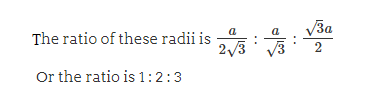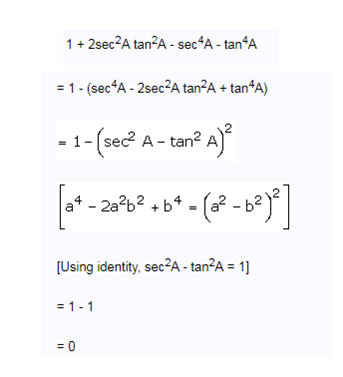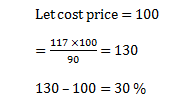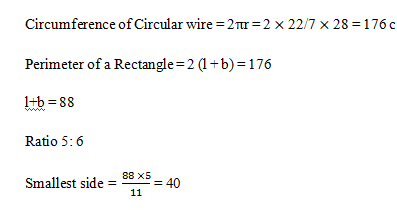# RRB ALP 2018 Practice Test Papers | Arithmetic Questions (Day-65)

Dear Aspirants, Here we have given the Important RRB ALP & Technicians Exam 2018 Practice Test Papers. Candidates those who are preparing for RRB ALP 2018 can practice these Arithmetic Questions to get more confidence to Crack RRB 2018 Examination.

[WpProQuiz 2902]

Click “Start Quiz” to attend these Questions and view Solutions

1) What are the ratio measures of the in-radius, circum-radius and one of the ex-radius of an equilateral triangle?

a) 1 : 2 : 5

b) 1 : 3 : 5

c) 1 : 2 : 3

d) 1 :√2: 2

2) In a bag, there are coins of 25 p, 50 p and 10 p in the ratio of 1:2: 3. If there is Rs. 62 in all, how many 50 p coins are there?

a) 120

b) 100

c) 80

d) 150

3) Find the value of 1 + 2sec2A.tan2A – sec4A – tan4A

a) √2

b) 2

c) 0

d) 1

4) Find the expression value

0.09 + 0.909 + 0.009 + 9.009 =?

a) 10.015

b) 10.018

c) 10.017

d) 10.016

5) 10 Men can complete a job in 12 days by working8 hours a day. 15 women working 16 days in 6 hours a day complete the same work. What is the ratio of efficient of Women and Men?

a) 4: 1

b) 1: 4

c) 2: 3

d) 3:2

6) A shopkeeper allows a discount of 10% on a selling price whereas he gains 17% profit of cost price. How much more Percent he marked on his cost price?

a) 20 %

b) 30 %

c) 50 %

d) 40 %

7) (80% of 750 + 72196) ÷5 = ?

a) 130

b) 127

c) 147

d) 154

8) A circular wire of radius28 cm is cut and bent in the form of a rectangle whose sides are in the ratio of 5 : 6. The small side of the rectangle is

a) 50

b) 44

c) 40

d) 60

9) Find the Next term

51975, 4725, 525, 75, ?, 5

a) 45

b) 35

c) 25

d) 15

10) Find the 3rd proportion of 36, 12.

a) 2

b) 6

c) 4

d) 5Let the number of 25 p, 50 p and 10 p coins be x, 2x, 3x respectively

Sum of values = Rs. (25x /100 + 100x /100 + 30x /100) = Rs. (155x /100)

62 =155 x /100

x = 40

∴Hence, the number of 50 p coins = (2 × 40) = 800.09 + 0.909 + 0.009 + 9.009 = 10.017

M1D1H1:W2D2H2

10×12×8: 15×16×6

Men : Women

3: 251975÷ 11 = 4725

4725÷9 =525

525÷ 7 =75

75÷5 = 15

15÷ 3 = 5